Navigation Panel:(These buttons explained below)Question Corner and Discussion Area

# Why is x^0 = 1?

Asked by Deliakos Argiris, T. E. I. (in Greece) on October 13, 1996:
Sorry for my English.

I do not understand why we agree with the axiom :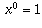.

When b is a positive integer,is defined to be the product of a multiplied by itself b times.

The question is, what is the most natural way to extend this definition to the case when b=0? Here are several ways to see that the definition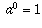is the only reasonable one:

1. Exponentiation satisfies the laws of exponents:. If we want this law to still be satisfied when we extend to the case b=0, we need to have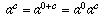, and therefore we need to have.

2. Ifis b copies of the number a, all multiplied together, then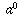should be the "empty product" with no factors multiplied together. In mathematics, the empty product is defined to be 1, because multiplying by nothing at all is the same as multiplying by 1.

3. Notice thatcan be thought of as "start with the number 1, then multiply by a, b times." For instance,and. Therefore,should be just 1, not multiplied by anything else at all.

4. When a is a positive integer, yet another reason for definingis thatis the number of ways of writing (in order) b numbers, each from 1 to a. For instance,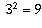because there are nine different pairs of numbers each of which is in the range from 1 to 3 (they are (1,1), (1,2), (1,3), (2,1), (2,2), (2,3), (3,1), (3,2), and (3,3)).

Therefore,should be the number of ways of writing no numbers, each of which is from 1 to a. There is exactly one way of doing this, namely, don't write any numbers at all!

(This reason is more compelling if you make it more mathematically precise, using the fact thatis the number of functions from a b-element set B to an a-element set A, and when b=0 the set B is the empty set, and there is exactly one function from the empty set into A, namely, the empty function).

The above reasons all illustrate why definingto be 1 is the only reasonable definition.

There's one other point worth mentioning: some of the reasons above are less compelling when a=0. For instance, in the first reason, we need to have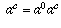, and if a is non-zero we can divide byto deduce that. However, if a=0 we no longer get a reason forto be 1.

Some of the reasons are still compelling, and, especially if we are in a context where only integer exponents are being considered, we still normally define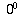to be 1.

However, if we define a two-variable function, then this function does not have a well-defined limit as (x,y) -> (0,0). We can define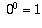if we like, but the limit still won't exist. In other words, if A and B each approach zero, there's no guarantee as to what (if anything)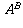approaches. It need not approach our definition of.

That's why, in calculus,is often called an indeterminate form. If one is working in situations where the exponent can continuously vary, it is usually better to leaveundefined to avoid making mistakes. However, if one is working in situations in which the exponent is always integral,is usually defined to be 1.

These complications are only for. When a is nonzero,is always defined to be 1, for the reasons given above.

[ Submit Your Own Question ] [ Create a Discussion Topic ]

This part of the site maintained by (No Current Maintainers)
Last updated: April 19, 1999
Original Web Site Creator / Mathematical Content Developer: Philip Spencer
Current Network Coordinator and Contact Person: Joel Chan - mathnet@math.toronto.edu

Navigation Panel: